Custom Search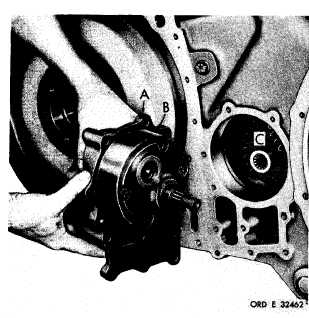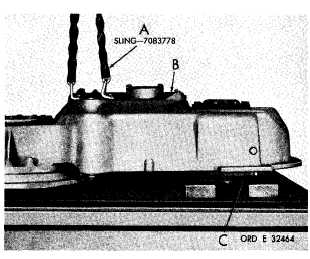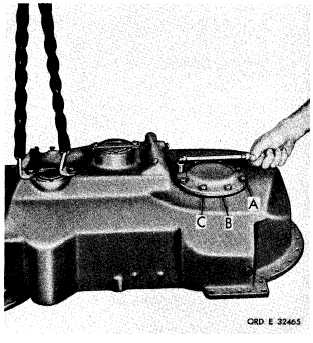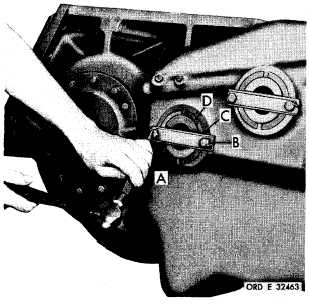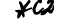*C2 P A R   74,   S T E P S   5 - 8 D I S A S S E M B LY C H A P   5,   SEC   II Figure   43   (Step   5) Remove   input   and   scavenge   oil   pump   assembly (A)   and   gasket   (B).   Remove   seal   ring   (C)   from input   transfer   housing   assembly. Figure   44   (Step   6) Straighten   corners   of   lock   strip   (A).   Using   a 9 / 1 6 - i n c h    w r e n c h ,    r e m o v e    t w o    b o l t s    ( B ) .    R e- m o v e    l o c k    s t r i p    ( A )    a n d    s t r a p    ( C )    r e t a i n i ng idler   cluster   gear   spindle   (D). Figure   45   (Step   7) Using   two   3/8-16   bolts   and   flat   washers,   at- tach   sling   (A)   to   input   transfer   housing   (B). P o s i t i o n    t h e    h o u s i n g    a s s e m b l y    o n    t h e    d i s - a s s e m b l y    t a b l e ,    s u p p o r t i n g    t o r q u e    c o n v e r t er (C),   and   keeping   the   transfer   housing   assembly l e v e l. Figure   46   (Step   8) Using   a   9/16-inch   wrench,   remove   eight   bolts and   lock   washers   (A)   from   converter   bearing cover   (B).   Remove   cover   (B)   and   gasket   (C). 7 9Integrated Publishing, Inc. - A (SDVOSB) Service Disabled Veteran Owned Small Business